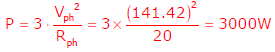# Power Electronics - Online Test

Q1. Circuit turn-off time of an SCR is defined as the time
Explaination / Solution:
No Explaination.

Q2. A three-phase current source inverter used for the speed control of an induction motor is to be realized using MOSFET switches as shown below. Switches S1 to S6 are identical switches.The proper configuration for realizing switches S1 to S6 is
Explaination / Solution:
No Explaination.

Q3. The transistor used in the circuit shown below has a β of 30 and ICBO is negligible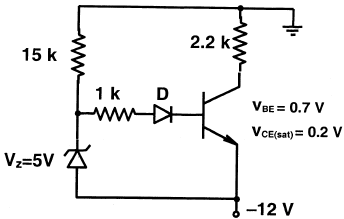If the forward voltage drop of diode is 0.7V, then the current through collector will be
Explaination / Solution:Q4. A voltage commutated chopper circuit, operated at 500Hz, is shown below.If the maximum value of load current is 10A, then the maximum current through the main (M) and auxiliary (A) thyristors will be
Explaination / Solution:Q5. A clipper circuit is shown below.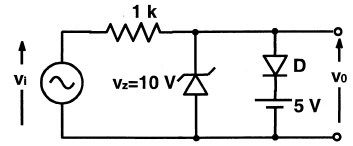Assuming forward voltage drops of the diodes to be 0.7V, the input-output transfer characteristics of the circuit is
Explaination / Solution: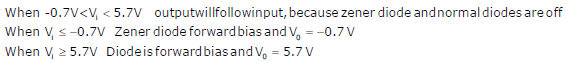Q6. The typical ratio of latching current to holding current in a 20 A thyristor is
Explaination / Solution:
No Explaination.

Q7. A half-controlled single-phase bridge rectifier is supplying an R-L load. It is operated at a firing angle α and the load current is continuous. The fraction of cycle that the freewheeling diode conducts is
Explaination / Solution:
No Explaination.

Q8. The i-v characteristics of the diode in the circuit given below are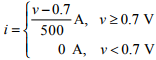The current in the circuit is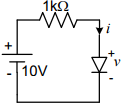Explaination / Solution: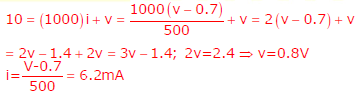Q9. In the 3-phase inverter circuit shown, the load is balanced and the gating scheme is 1800 -conduction mode. All the switching devices are ideal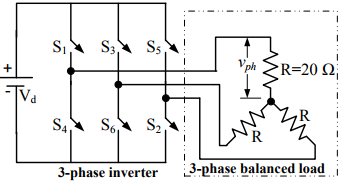The rms value of load phase voltage is
Explaination / Solution:Q10. In the 3-phase inverter circuit shown, the load is balanced and the gating scheme is 1800 -conduction mode. All the switching devices are idealIf the dc bus voltage V= 300 V, the power consumed by 3-phase load is
Explaination / Solution: